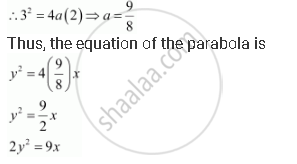CBSE (Arts) Class 11CBSE
Share

# Find the Equation of the Parabola that Satisfies the Following Conditions: Vertex (0, 0) Passing Through (2, 3) and Axis is Along X-axis - CBSE (Arts) Class 11 - Mathematics

ConceptParabola Standard Equations of Parabola

#### Question

Find the equation of the parabola that satisfies the following conditions: Vertex (0, 0) passing through (2, 3) and axis is along x-axis

#### Solution

Since the vertex is (0, 0) and the axis of the parabola is the x-axis, the equation of the parabola is either of the form y2 = 4ax or y2 = –4ax.

The parabola passes through point (2, 3), which lies in the first quadrant.

Therefore, the equation of the parabola is of the form y2 = 4ax, while point

(2, 3) must satisfy the equation y2 = 4ax.Is there an error in this question or solution?

#### Video TutorialsVIEW ALL 

Solution Find the Equation of the Parabola that Satisfies the Following Conditions: Vertex (0, 0) Passing Through (2, 3) and Axis is Along X-axis Concept: Parabola - Standard Equations of Parabola.
S